# What Does “if __name__ == ‘__main__'” Do in Python?

Rate this post

Today, let’s discuss something that’s all over the place in many code bases: what does `if __name__ == '__main__'` do in Python?

The statement `if __name__ == '__main__':` checks if variable `__name__` is set to the string value `'__main__'` which holds only within the main source file from which you initially execute your code. In all other contexts—such as in imported code files—the variable `__name__` is set to a different value.

Thus, `if __name__ == '__main__':` evaluates to `True` if you use it in your main file (the one you run with `python main.py`). If you import it indirectly, it evaluates to `False`.

This is useful because you usually don’t want to run code with side effects when importing modules.

You can play with the code in this minimal example:

Exercise: Run the code shell. What’s the output? Now, create a new file and `import main`. What’s the output now?

If you are learning Python programming step by step, you would have bumped into the above piece of code snippet somewhere. Even if you have not, I am sure you will soon come across it, and this might feel like a jerk in your so far smooth journey of learning Python programming. I have been there. I was going through a book on Python programming when I first saw this piece of code, and I thought—wait a minute, did I miss anything on my way up to here? Then I went back, revising the last few pages to figure out what I missed, and this loop went on for a while before I finally sat down to face the demon, and honestly, I smiled when I figured it out, it is that simple. Maybe, we learn this way. We go back and forth, keep jumping, and then one day, the magic happens. The only thing that works is not giving up.

Today I will try to make this understanding simple for you in a way that will hopefully bring a smile to your face if you have been in the loop of getting a hint of what it is and then keep forgetting.

To understand what is `if __name__ == __main__` used for we will dive into some code examples first to understand what `__name__` and `__main__` are. Then we will cement the learning through another example. So be patient and go through the article carefully. The code snippets may look a little confusing but wait until we explain and see what is happening exactly.

## What are `__name__` and `__main__`?

Let us start with `__name__`. Simply put, `__name__` is a special variable that is built-in in Python.

In Python, we can run code as a source file or, in other words, a python code that runs on its own (read – not imported from some separate Python source file). This sentence may be confusing at the moment, but let us explore this further.

Look at the code snippet below. It is a simple python file with just one line:

`print(f" First Module's Name : {__name__}")`

Simply put, we are asking Python to print the value that `__name__` variable has at this moment. Notice there is no import statement or even any other line of code above it.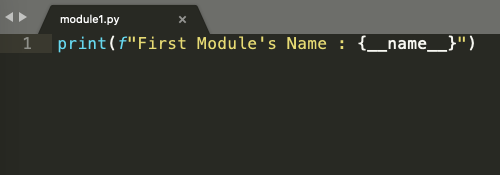Figure 1

Check the output in Figure 2 below. We run the above lines of code and find the output

First Module’s Name : `__main__`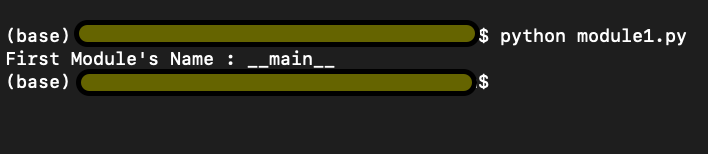Figure 2

We import `module1.py` into `module2.py` and ask Python to return the second module’s name (second because this is the second python code we are running) stored in` __name__` variable again.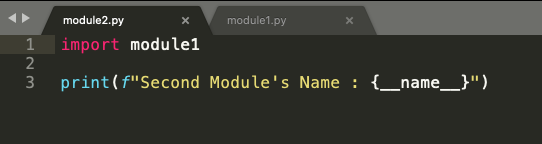Figure 3

```# File: module2.py
import module1

print(f"Second Module's Name : {__name__}")```

Interestingly, in Figure 5 below, we have the output

First Module’s Name : module1

Second Module’s Name : __main__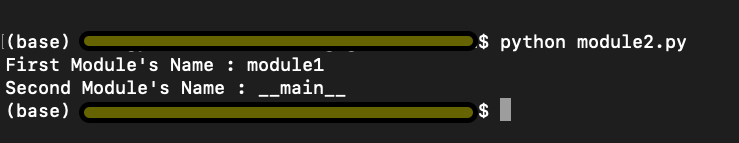Figure 4

The above information summarised simply can be explained as follows. Python starts executing the first line of code found in a Python file, but before it runs anything to show the output, Python defines some special variables. __name__ is one such variable. When the source file is executed on its own i.e, not being imported from some other source code, the __name__ variable is set to value __main__, and hence the output we got in Figure 3 was First Module’s Name : __main__. If you import the file from some other module (as in the code snippet from Figure 4), the value of __name__ variable is set to the name of the module it is imported from. Hence the output in Figure 5.

Now let us look at the sequence of execution.

1. module1.py in Figure 1 is executed,
2. Variable __name__ is set to the value __main__
3. __main__ module is executed, and the output is First Module’s Name : __main__
4. Module2.py in Figure 4 is executed,
5. Variable __name__ is set to the value module1
6. module1 is the block of code in module1.py which is executed, and the output is First Module’s Name : module1
7. Next, we move on to line 3 in module2.py, the name value is reset to __main__, because this is a fresh piece of code in module2.py, and the output is Second Module’s Name : __main__

## Using if __name__ == ‘__main__’

But let’s think only concerning the code in module2.py. In the real world, with module2.py I would not like to print the output from module1.py rather only import some functionality and get output for the lines of code written in module2.py i.e. Second Module’s Name : __main__

But how can we do it? There has to be a way because this seems more logical that way. Refer to the code snippet below in Figure 6.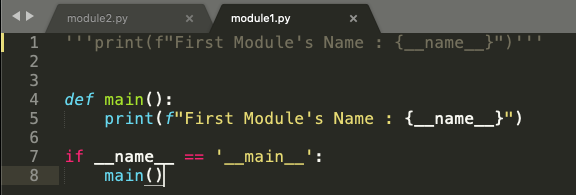Figure 5

```# File: module1.py
'''print(f"First Module's Name : {__name__}")'''

def main():
print(f"First Module's Name : {__name__}")

if __name__ == '__main__':
main()```

So, we will define a function `main()`, and put the print statement from `module1.py` inside the main function. Now we use this small trick of using the statement `if __name__ == '__main__'` and put the main function inside this if statement. Now let’s look at the execution sequence of our program.

1. We have a `main()` function where we ask to print the name of the first module stored in the `__name__` variable. From the above example, we know the value stored in the `__name__` variable is `__main__`.
2. In line 7, we say if the value in `__name__` variable is equal to `__main__`, only then go ahead and execute the `main()` function.
3. Now running the modified module1.py, we can see the output as First Module’s Name : `__main__` as expected. Refer to the first output in Figure 7
4. Now when we run module2.py, we get the output as Second Module’s Name : `__main__`

Notice how the output for module2.py has changed now. Although we have imported module1 in our module2.py program, the program in module1.py doesn’t run. Why? Because the value stored in the __name__ variable at this moment is module1 and not __main__. So, using a main() function and if __name__ == ‘__main__’ has put restrictions on execution of lines of code in module1.py as it would only execute module1.py only if __name__ == ‘__main__’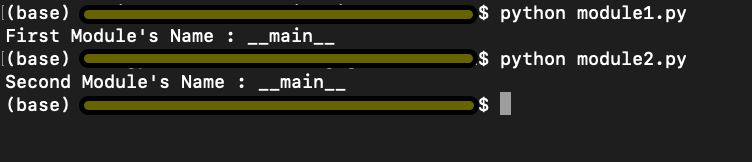Figure 6

## Putting it all into a real-world example

We now take a more generic example, which would make more sense of all we have discussed above.

• Have a look at the below code snippet from `cubes.py` in Figure 8. In line 1 and line 2, we have a function `cube()`, which takes `num` as an argument. The function `cubes(num)` returns the cube of a given number.
• On line 4, we use a for loop which iterates over a list of numbers from 0 to 9.
• On line 5, we use the `cubes(num)` function with i as input to display the cube, as shown in figure 9. Simple enough.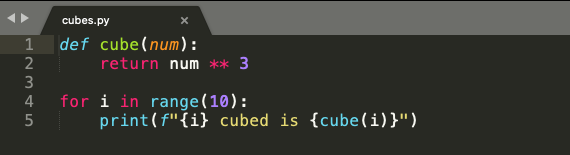Figure 7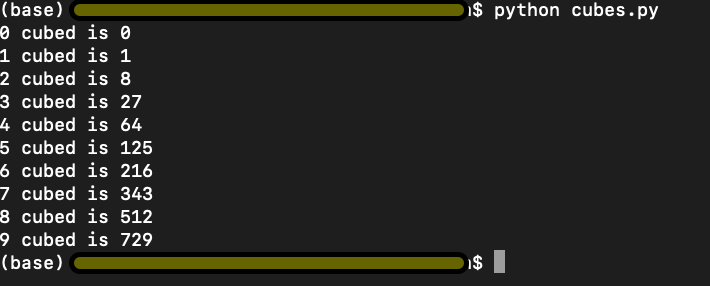Figure 8

```def cube(num):
return num ** 3

for i in range(10):
print(f"{i} cubed is {cube(i)}")```

In the code snippet from `modules.py` below in Figure 10, we import `cube(num)` function from `cubes.py` and try to print cube of 10 in line 3. Logically, we are trying to use the function `cube(num)`, which returns a cube of any given input number.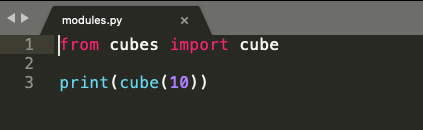Figure 9

```# File modules.py
from cubes import cube

print(cube(10))```

However, look at the output below. We have the output of both the programs `cubes.py` and `modules.py`. This is not what we intended or wanted to do.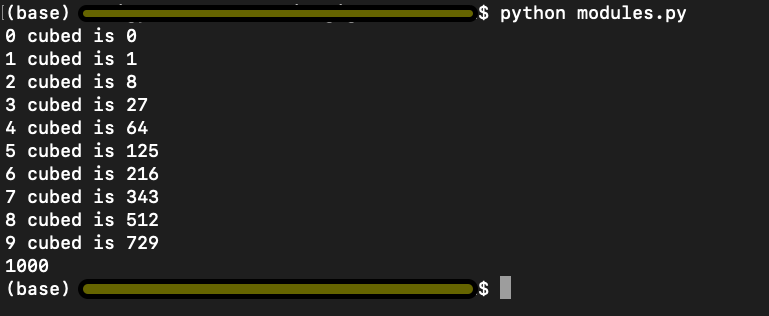Figure 10

Remember the explanation from above where we used if __name__ == ‘__main__’ to limit the execution of code from `modules1.py`. Let us try and implement the same idea and see if that works here.

In the code snippet from `cubesModified.py` in Figure 11, we put the for loop and the print statement from cubes.py in the main() function. And again, we use the statement if __name__ == ‘__main__’ to execute code from cubesModified.py only when the value of __name__ variable equals to __main__.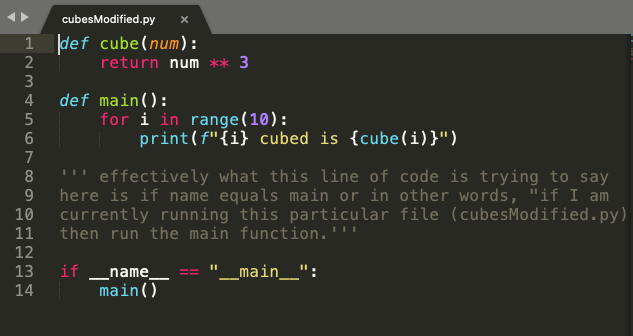Figure 11

```# File: cubesModified.py
def cube(num):
return num ** 3

def main():
for i in range(10):
print(f"{i} cubed is {cube(i)}")

''' effectively what this line of code is trying to say
here is if name equals main or in other words, "if I am
currently running this particular file (cubesModified.py)"
then run the main function.'''

if __name__ == "__main__":
main()```

We run `cubesModified.py` and get the output as below and per our expectation.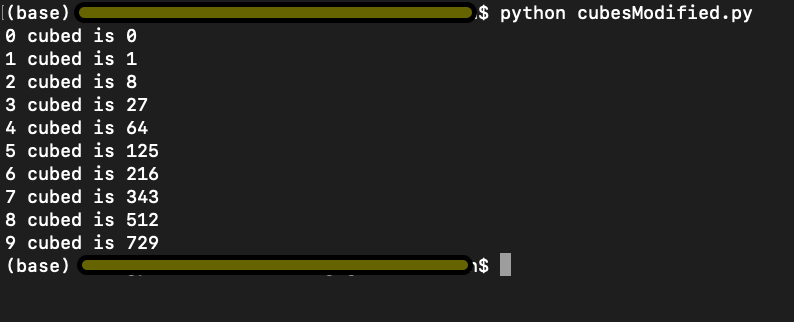Figure 12

However, now when we run `modulesModified.py` where we import `cube(num)` function from `cubesModified.py`, it only executes the code from our `modulesModified.py` as shown in Figure 15, and the output is 1000 because the value stored in the __name__ variable in cubesModified and not __main__.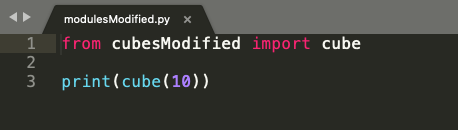Figure 13

```# File: cubesModified
from cubesModified import cube

print(cube(10))```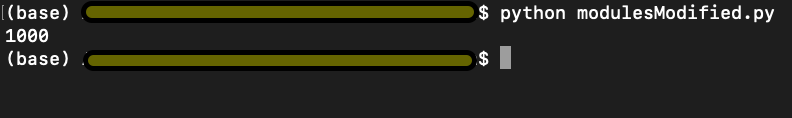Figure 14

I hope this explanation helps you with a better understanding of what does if __name__ == ‘__main__’. Go ahead and play around with the code in the code editor and cement your understanding. Best of Luck!!